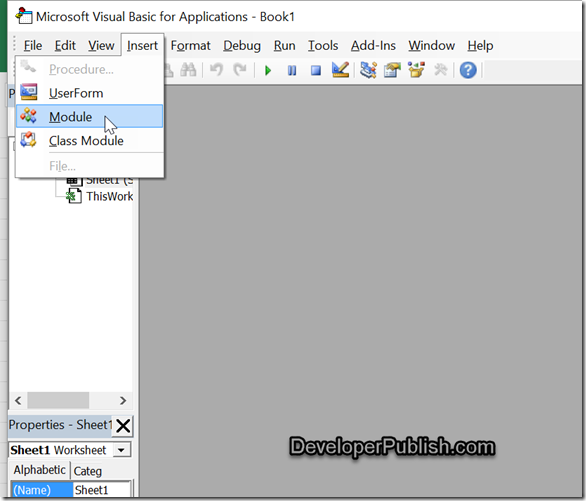# Removing Non numeric characters from cell in Microsoft Excel using VBA

This blog will demonstrate how to remove the non numeric characters from the cell in Microsoft Excel 2016.

Assume that you have a worksheet where a cells from the range “A1:D30” contains the alpha-numeric characters like ABHGFD458909LOGH and you have a task to retain only the numeric characters in the cell and remove the non-numeric characters.

# How can you remove the non-numeric characters from a cell in Microsoft Excel ?

One of the options we have is to use the the VBA code to remove the non-numeric characters.

1. Open the Microsoft Visual Basic for Application Window using the shortcut key “ALT + F11”.

2. In the Microsoft Visual Basic for Application Window , select the menu Insert –> Module.3. Paste the following code in the Module window and press F5 key to run it.

```Sub RemoveNonNumeric()
Dim sheetName As String
sheetName = "Sheet1"
Dim CellRange As String
CellRange = "A1:D30"
Dim rng As Range
For Each rng In Sheets(sheetName).Range(CellRange).Cells
Dim index As Integer
Dim result As String
result = ""
For index = 1 To Len(rng.Value)
Select Case Asc(Mid(rng.Value, index, 1))
Case 48 To 57:
result = result & Mid(rng.Value, index, 1)
End Select
Next
rng.Value = result
Next
End Sub
```

You should immediately see only the numeric characters in the cell…